Function Repository Resource:

# ConicAnamorphosisMap

Convert a point reflected in the base of a right conical mirror to its conic anamorphic map in the xy plane

Contributed by: Erik Mahieu
 ResourceFunction["ConicAnamorphosisMap"][pt,α] converts a point pt inside the unit disk base of a right circular cone with opening angle α to its conical anamorphic map in the xy-plane.

## Details and Options

The domain of ResourceFunction["ConicAnamorphosisMap"] is the unit disk Disk[].
The range of ResourceFunction["ConicAnamorphosisMap"] is Annulus[{0,0},{1,Tan[α]/Tan[α/2]] with α<Pi/2.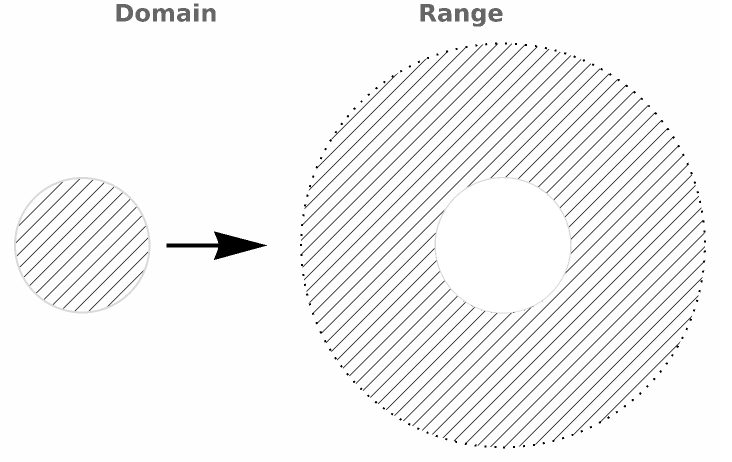## Examples

### Basic Examples (1)

Get the anamorphic map of a point reflected in the base of a cone with a 60 Degree opening angle:

 In:=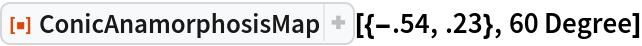Out=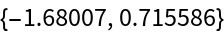### Scope (1)

The function can be applied to sets of points as e.g. a 5 star polar curve: the blue curve is the reflected curve and the red curve is the conical anamorphic curve:

 In:=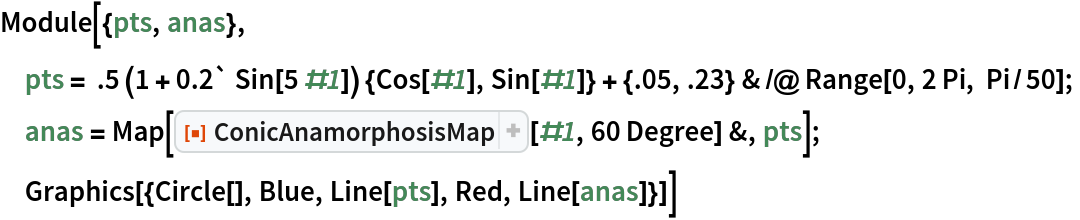Out=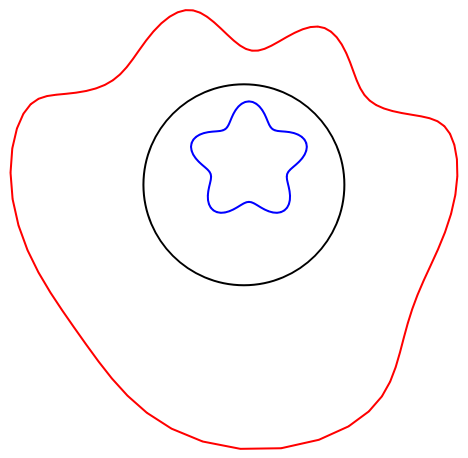### Applications (1)

The function can be applied to photographic images that are converted into a radial set of colored polygons:

 In:=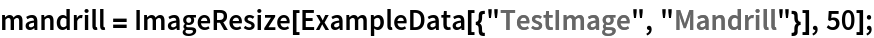In:=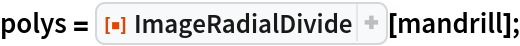In:=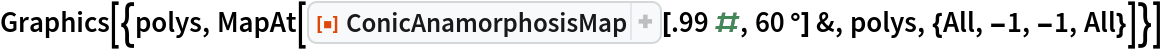Out=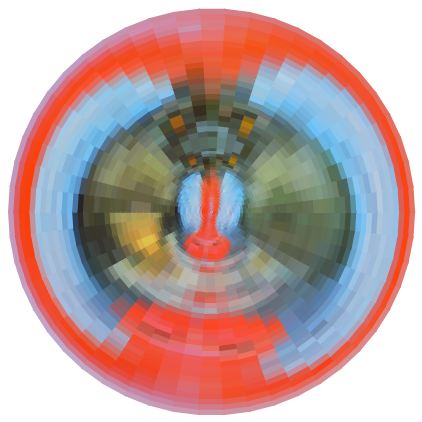### Neat Examples (1)

Circular logos are ideally suited for conical anamorphic images. Here are a top (right) and default 3D view of an anamorphic conversion of the Wolfram logo reflected in a conical mirror:

 In:=In:=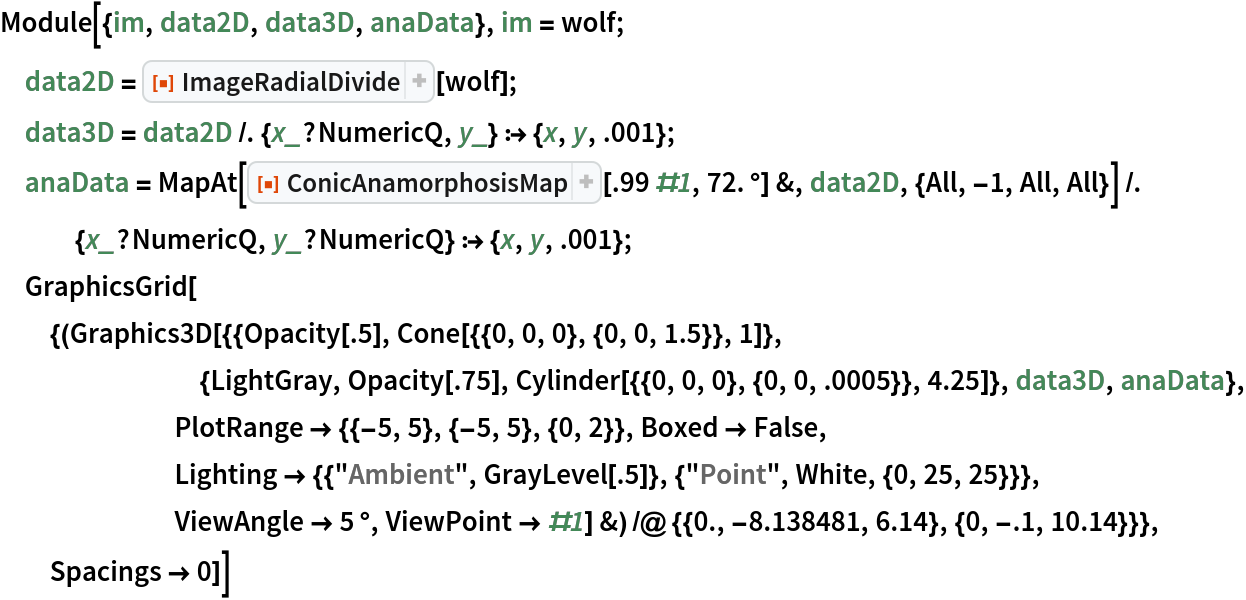Out=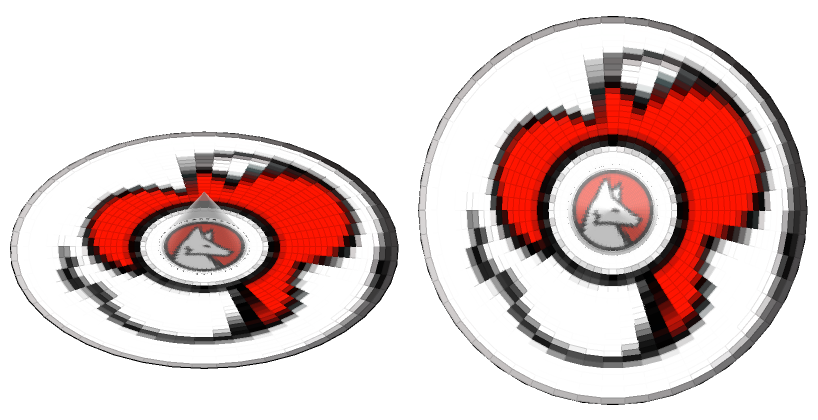Erik Mahieu

## Version History

• 1.0.0 – 23 November 2020